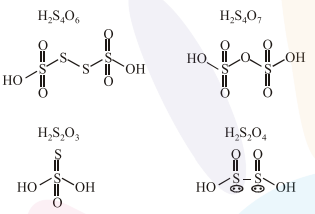# The oxoacid of sulphur that does not contain bond between sulphur atoms is :`
Question:

The oxoacid of sulphur that does not contain bond between sulphur atoms is :

1. $\mathrm{H}_{2} \mathrm{~S}_{4} \mathrm{O}_{6}$

2. $\mathrm{H}_{2} \mathrm{~S}_{2} \mathrm{O}_{7}$

3. $\mathrm{H}_{2} \mathrm{~S}_{2} \mathrm{O}_{3}$

4. $\mathrm{H}_{2} \mathrm{~S}_{2} \mathrm{O}_{4}$

Correct Option: , 2

Solution:$\mathrm{H}_{2} \mathrm{~S}_{2} \mathrm{O}_{7}$ does not contain bond between sulphur atoms.